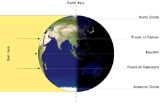xEquinoxOverview

An equinox occurs twice a year, when the tilt of the Earth
Earth
Earth is the third planet from the Sun, and the densest and fifth-largest of the eight planets in the Solar System. It is also the largest of the Solar System's four terrestrial planets...

's axis is inclined neither away from nor towards the Sun
Sun
The Sun is the star at the center of the Solar System. It is almost perfectly spherical and consists of hot plasma interwoven with magnetic fields...

, the center of the Sun being in the same plane as the Earth's equator
Equator
An equator is the intersection of a sphere's surface with the plane perpendicular to the sphere's axis of rotation and containing the sphere's center of mass....

. The term equinox can also be used in a broader sense, meaning the date when such a passage happens.
The name "equinox" is derived from the Latin aequus (equal) and nox (night), because around the equinox, the night and day have approximately equal length.

At an equinox, the Sun is at one of two opposite points on the celestial sphere
Celestial sphere
In astronomy and navigation, the celestial sphere is an imaginary sphere of arbitrarily large radius, concentric with the Earth and rotating upon the same axis. All objects in the sky can be thought of as projected upon the celestial sphere. Projected upward from Earth's equator and poles are the...

where the celestial equator
Celestial equator
The celestial equator is a great circle on the imaginary celestial sphere, in the same plane as the Earth's equator. In other words, it is a projection of the terrestrial equator out into space...

(i.e.